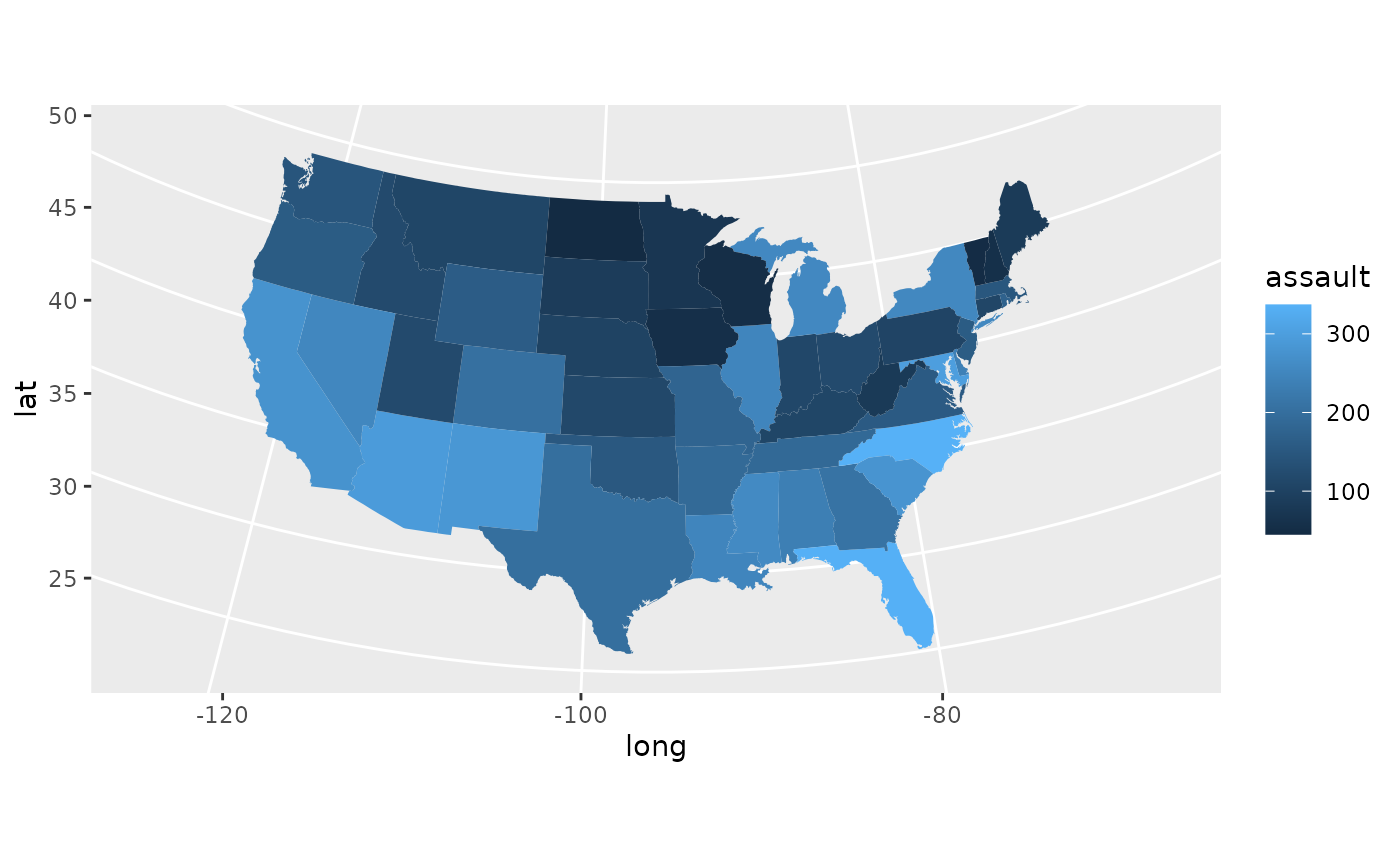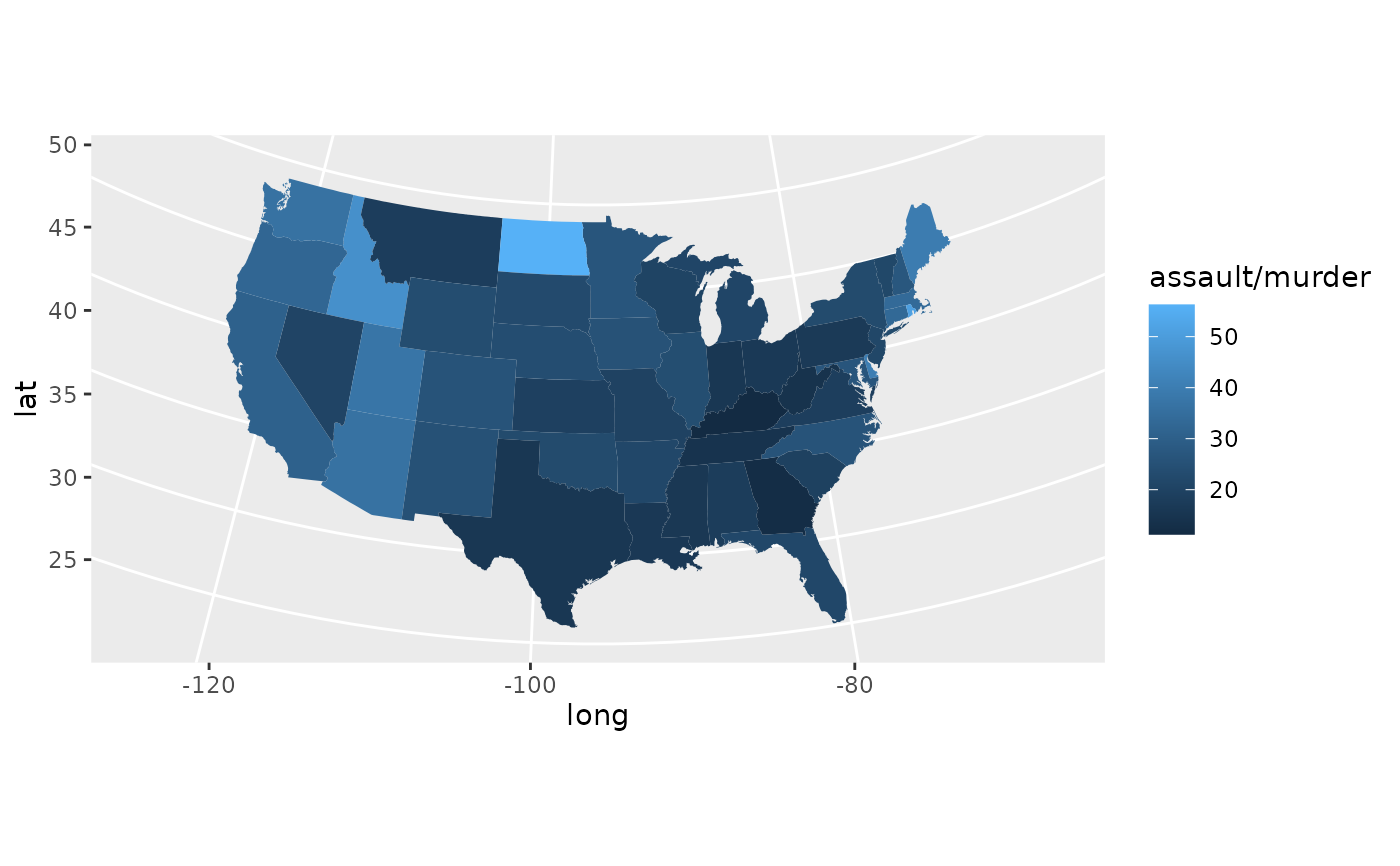Easily turn data from the maps package into a data frame suitable for plotting with ggplot2.

## Usage

map_data(map, region = ".", exact = FALSE, ...)

## Arguments

map

name of map provided by the maps package. These include maps::county(), maps::france(), maps::italy(), maps::nz(), maps::state(), maps::usa(), maps::world(), maps::world2().

region

name(s) of subregion(s) to include. Defaults to . which includes all subregions. See documentation for maps::map() for more details.

exact

should the region be treated as a regular expression (FALSE) or as a fixed string (TRUE).

...

all other arguments passed on to maps::map()

## Examples

if (require("maps")) {
states <- map_data("state")
arrests <- USArrests
names(arrests) <- tolower(names(arrests))
arrests$region <- tolower(rownames(USArrests)) choro <- merge(states, arrests, sort = FALSE, by = "region") choro <- choro[order(choro$order), ]
ggplot(choro, aes(long, lat)) +
geom_polygon(aes(group = group, fill = assault)) +
coord_map("albers",  lat0 = 45.5, lat1 = 29.5)
}if (require("maps")) {
ggplot(choro, aes(long, lat)) +
geom_polygon(aes(group = group, fill = assault / murder)) +
coord_map("albers",  lat0 = 45.5, lat1 = 29.5)
}